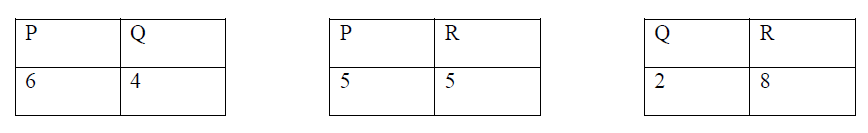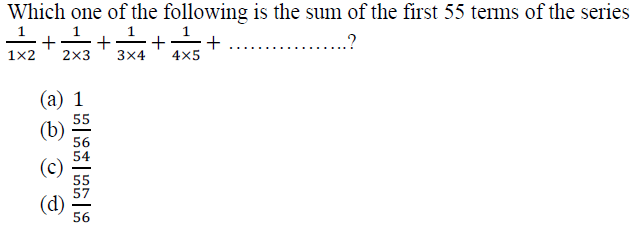### Solved Question Paper for Stores Officer in Geological Survey of India conducted by UPSC

31. Three teams P, Q and R played against each other equal number of matches. The following Tables show the number of wins:Which one of the following statements is correct?

(a) P lost 55% of the matches

(b) Q lost 70% of the matches

(c) R won 35% of the matches

(d) Q won 20% of the matches

32. There are two types of rice. Type I is sold for Rs. 10 per Kg whereas type II is sold for Rs. 100 per Kg. A seller mixed these two types of rice and sells for Rs. 80 per Kg. If there is no change in the profit of the seller, then proportion of the two types of rice is:

(a) 1 : 1

(b) 7 : 9

(c) 2 : 7

(d) 9 : 16

33.34. In a 1000 meters race, ‘A’ beats ‘B’ by 35 meters or 7 seconds. Which one of the following was A’s timing over the course?

(a) 200 seconds

(b) 196 seconds

(c) 193 seconds

(d) 190 seconds

35. The cost price of an article is Rs. 100. If the article is sold at a discount of 18% and then there is a tax of 18%, what is the selling price of the article including tax?

(a) Rs. 100.00

(b) Rs. 98.46

(c) Rs. 96.76

(d) Rs. 90.50

36. The number of ordered triplets of positive integers which are the solutions of the equation

x+y+z = 10 is:

(a) 49

(b) 36

(c) 25

(d) 16

37. In an examination ‘R’ got more marks than ‘M’ but not as many as ‘P’. ‘P’ got more marks than ‘G’ and ‘K’. ‘G’ got less marks than ‘M’ but his marks are not the lowest in the group. Who among the following is second in the descending order of marks?

(a) P

(b) K

(c) R

(d) Can’t be determined

38. In an examination, 43% passed in Mathematics, 48% passed in Economics and 52% passed in Statistics. Only 8% passed in all the three subjects. 14% passed in Mathematics and Economics and 21% passed in Mathematics and Statistics and 20% passed in Economics and Statistics. Number of examinees who took the examination is 300. The number of examinees who passed only one of the subjects is:

(a) 57

(b) 63

(c) 114

(d) 171

39. What is the number of numbers between 200 and 400 such that when they are divided by 6, 8 or 9 it leaves in each case a remainder 3?

(a) 1

(b) 2

(c) 3

(d) 4

40. ‘A’ can run 330 meters in 41 seconds and ‘B’ in 44 seconds. By how many seconds will ‘B’ win if ‘B’ starts from 30 meters?

(a) 1 second

(b) 2 seconds

(c) 3 seconds

(d) 4 seconds

Share:

## Featured Post

### UPSC Civil Service Preliminary Paper-1 Previous Year Solved Question Papers

Civil Service Preliminary Paper-1 Previous Year Solved Questions for the year 2019 Civil Service Preliminary Paper-1 Previous Year Solved Qu...Name

Email *

Message *